# How to Calculate and Solve for Discharge | Chezy’s Equation | Water Budget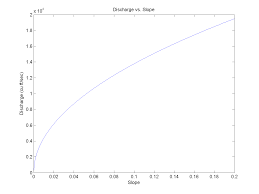The image above represents discharge | Chezy’s Equation.

To compute for discharge, four essential parameters are needed and these parameters are Area (A), Chezy’s Constant (C), Hydraulic Radius (R) and Slope (S).

The formula for calculating discharge:

Q = AC√(RS)

Where;

Q = Discharge
A = Area
C = Chezy’s Constant
S = Slope

Let’s solve an example;
Find the discharge when the area is 25, the chezy’s constant is 16, the hydraulic radius is 9 and the slope is 21.

This implies that;

A = Area = 25
C = Chezy’s Constant = 16
R = Hydraulic Radius = 9
S = Slope = 21

Q = AC√(RS)
Q = (25)(16)√((9)(21))
Q = (25)(16)√(189)
Q = (25)(16)(13.74)
Q = 5499.09

Therefore, the discharge according to Chezy’s equation is 5499.09.

Calculating the Area when the Discharge, the Chezy’s Constant, the Hydraulic Radius and the Slope is Given.

A = Q / C √RS

Where;

A = Area
Q = Discharge
C = Chezy’s Constant
S = Slope

Let’s solve an example;
Find the area when the discharge is 40, the chezy’s constant is 10, the hydraulic radius is 5 and the slope is 9.

This implies that;

Q = Discharge = 40
C = Chezy’s Constant = 10
R = Hydraulic Radius = 5
S = Slope = 9

A = Q / C √RS
A = 40 / 10 √(5)(9)
A = 40 / 10 √45
A = 40 / 10 (6.708)
A = 40 / 67.08
A = 0.596

Therefore, the area is 0.596.

Calculating the Chezy’s Constant when the Discharge, the Area, the Hydraulic Radius and the Slope is Given.

C = Q / A √RS

Where;

C = Chezy’s Constant
Q = Discharge
A = Area
S = Slope

Let’s solve an example;
Given that the discharge is 38, the area is 24, the hydraulic radius is 12 and the slope is 7. Find the Chezy’s Constant?

This implies that;

Q = Discharge = 38
A = Area = 24
R = Hydraulic Radius = 12
S = Slope = 7

C = Q / A √RS
C = 38 / 24 √(12)(7)
C = 38 / 24 √84
C = 38 / 24 (9.16)
C = 38 / 219.84
C = 0.172

Therefore, the chezy’s constant is 0.172.

Calculating the Hydraulic Radius when the Discharge, the Area, the Chezy’s Constant and the Slope is Given.

R = (Q / AC)2 / S

Where;

C = Chezy’s Constant
Q = Discharge
A = Area
S = Slope

Let’s solve an example;
Find the hydraulic radius when the chezy’s constant is 5, the discharge is 50, the area is 7 and the slope is 2.

This implies that;

C = Chezy’s Constant = 5
Q = Discharge = 50
A = Area = 7
S = Slope = 2

R = (Q / AC)2 / S
R = (50 / 7 x 5)2 / 2
R = (50 / 35)2 / 2
R = (1.428)2 / 2
R = 2.04 / 2
R = 1.02

Therefore, the hydraulic radius is 1.02.

Calculating the Slope when the Discharge, the Area, the Chezy’s Constant and the Hydraulic Radius is Given.

S = (Q / AC)2 / R

Where;

S = Slope
Q = Discharge
A = Area
C = Chezy’s Constant

Let’s solve an example;
Find the slope when the discharge is 80, the area is 10, the chezy’s constant is 4 and the hydraulic radius is 8.

This implies that;

Q = Discharge = 80
A = Area  = 10
C = Chezy’s Constant = 4
R = Hydraulic Radius = 8

S = (Q / AC)2 / R
S = (80 / 10 x 4)2 / 8
S = (80 / 40)2 / 8
S = (2)2 / 8
S = 4 / 8
S = 0.5

Therefore, the slope is 0.5.

Nickzom Calculator – The Calculator Encyclopedia is capable of calculating the discharge | chezy’s equation.

To get the answer and workings of the discharge | chezy’s equation using the Nickzom Calculator – The Calculator Encyclopedia. First, you need to obtain the app.

You can get this app via any of these means:

To get access to the professional version via web, you need to register and subscribe for NGN 1,500 per annum to have utter access to all functionalities.
You can also try the demo version via https://www.nickzom.org/calculator

Apple (Paid) – https://itunes.apple.com/us/app/nickzom-calculator/id1331162702?mt=8
Once, you have obtained the calculator encyclopedia app, proceed to the Calculator Map, then click on Agricultural under Engineering.Now, Click on Water Budget under AgriculturalNow, Click on Discharge| Chezy’s Equation under Water Budget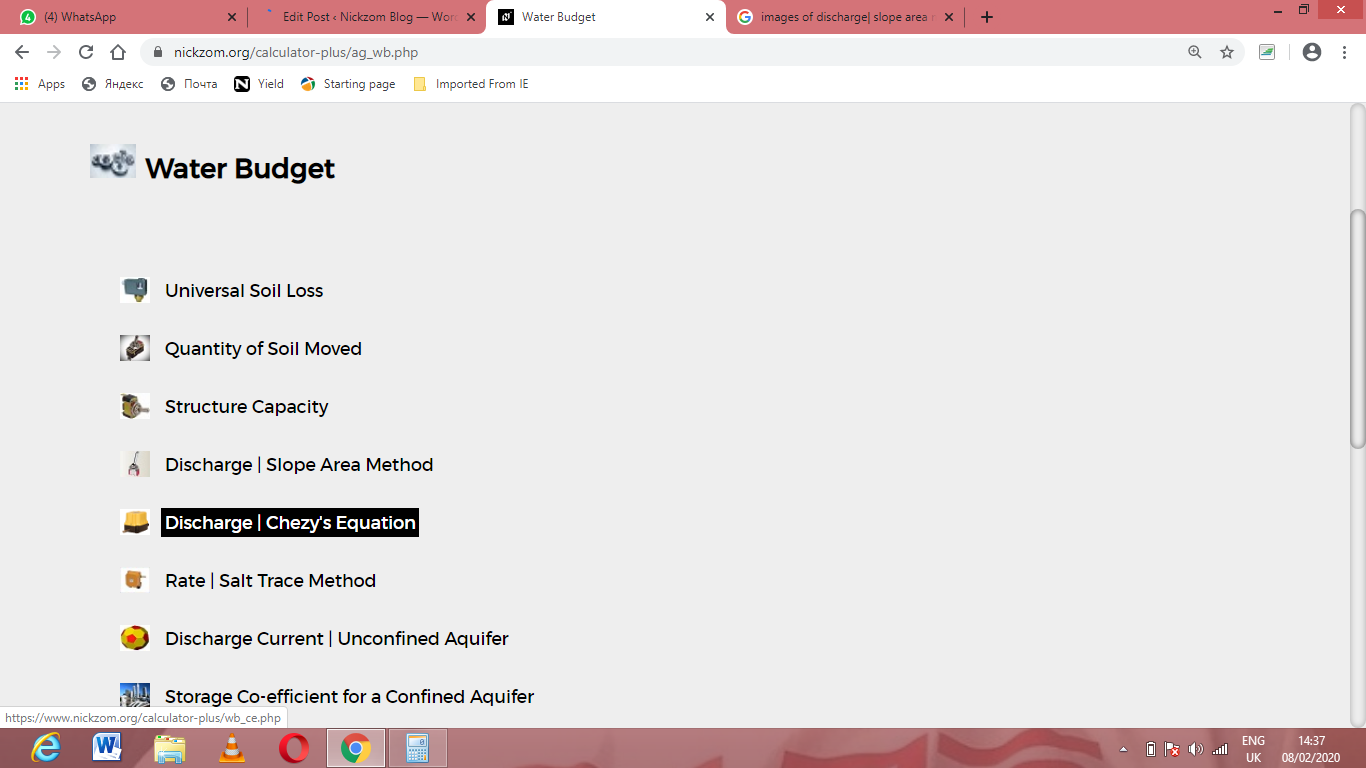The screenshot below displays the page or activity to enter your values, to get the answer for the discharge | chezy’s equation according to the respective parameters which are the Area (A), Chezy’s Constant (C), Hydraulic Radius (R) and Slope (S).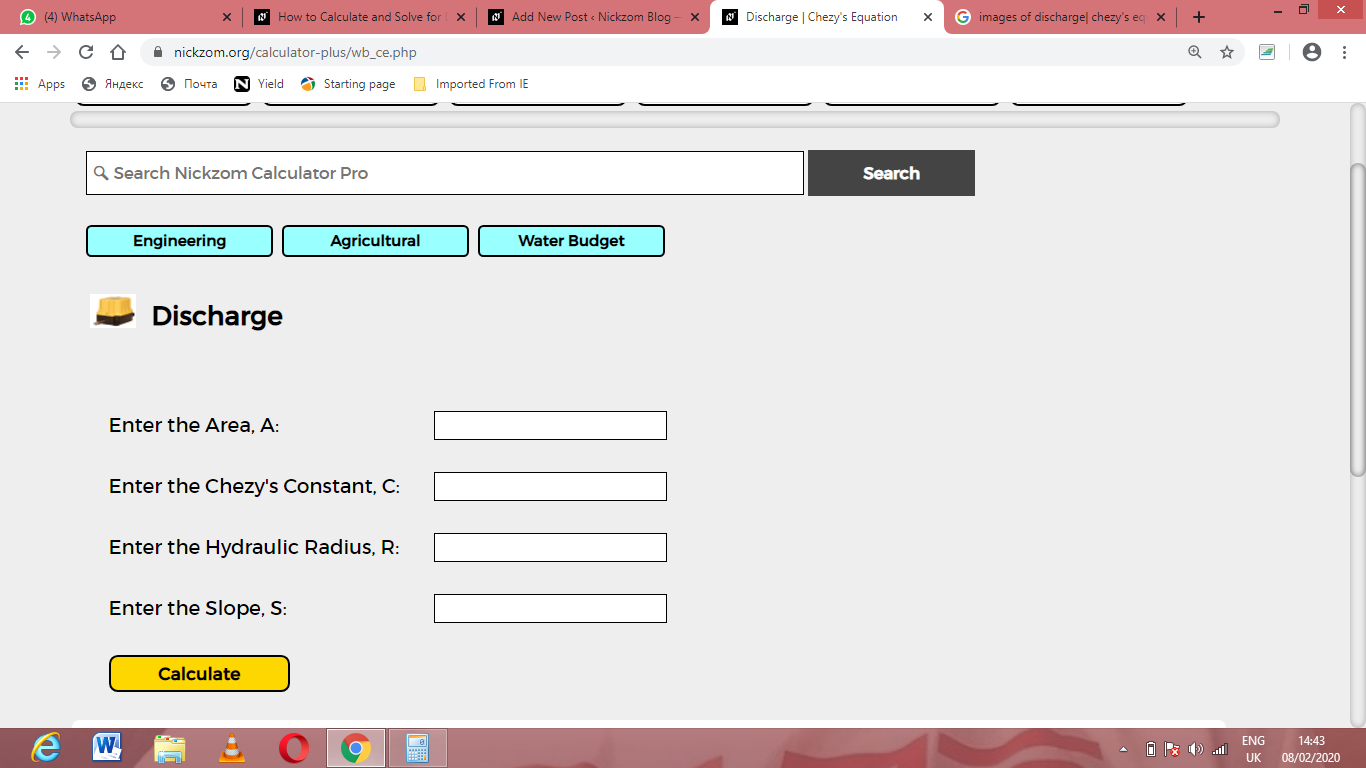Now, enter the values appropriately and accordingly for the parameters as required by the Area (A) is 25, Chezy’s Constant (C) is 16, Hydraulic Radius (R) is 9 and Slope (S) is 21.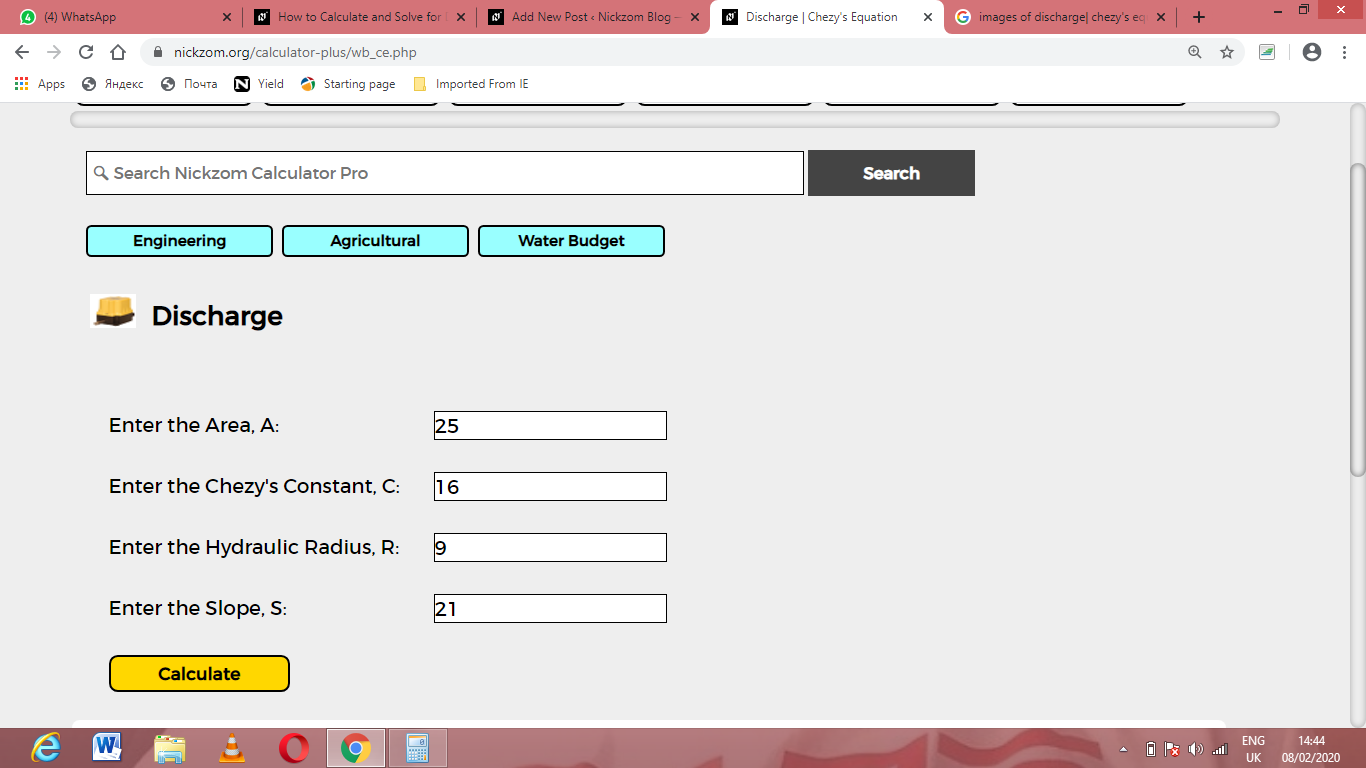Finally, Click on Calculate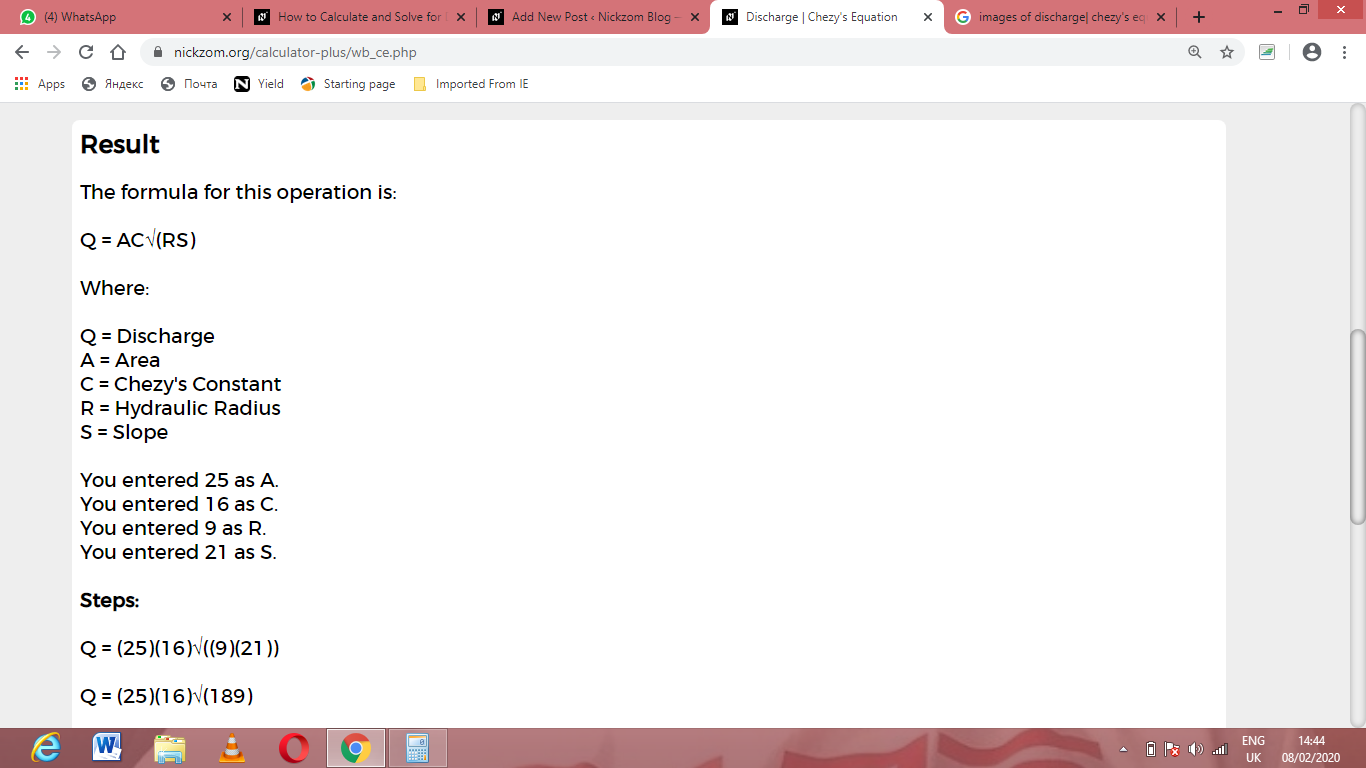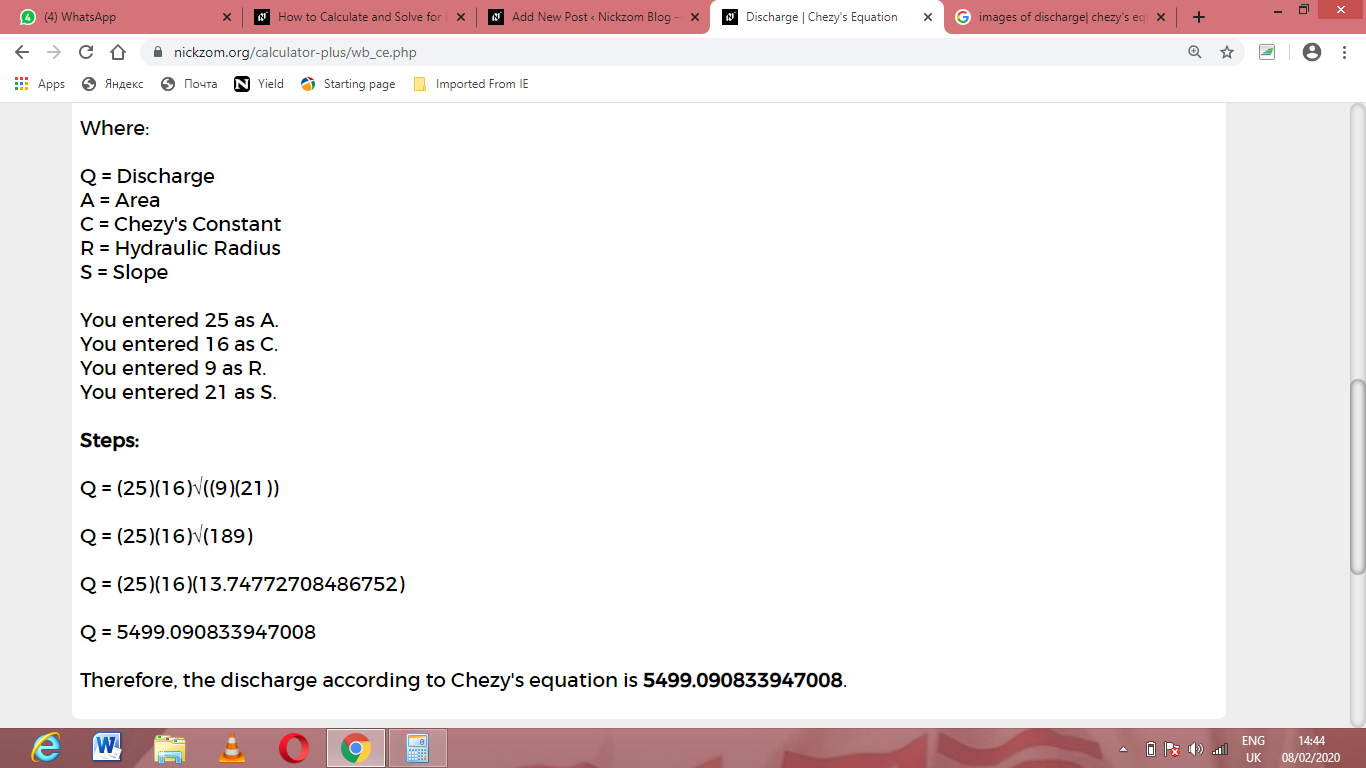As you can see from the screenshot above, Nickzom Calculator– The Calculator Encyclopedia solves for the discharge | chezy’s equation and presents the formula, workings and steps too.